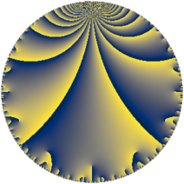# Properties

 Label 207.6.eLevel $207$ Weight $6$ Character orbit 207.e Rep. character $\chi_{207}(70,\cdot)$ Character field $\Q(\zeta_{3})$ Dimension $220$ Sturm bound $144$

# Related objects

## Defining parameters

 Level: $$N$$ $$=$$ $$207 = 3^{2} \cdot 23$$ Weight: $$k$$ $$=$$ $$6$$ Character orbit: $$[\chi]$$ $$=$$ 207.e (of order $$3$$ and degree $$2$$) Character conductor: $$\operatorname{cond}(\chi)$$ $$=$$ $$9$$ Character field: $$\Q(\zeta_{3})$$ Sturm bound: $$144$$

## Dimensions

The following table gives the dimensions of various subspaces of $$M_{6}(207, [\chi])$$.

Total New Old
Modular forms 244 220 24
Cusp forms 236 220 16
Eisenstein series 8 0 8

## Trace form

 $$220 q - 8 q^{2} + 22 q^{3} - 1760 q^{4} - 65 q^{6} - 58 q^{7} + 1194 q^{8} + 506 q^{9} + O(q^{10})$$ $$220 q - 8 q^{2} + 22 q^{3} - 1760 q^{4} - 65 q^{6} - 58 q^{7} + 1194 q^{8} + 506 q^{9} + 78 q^{11} - 3988 q^{12} + 362 q^{13} - 1010 q^{14} + 14 q^{15} - 28160 q^{16} + 4080 q^{17} + 15217 q^{18} - 1912 q^{19} - 826 q^{20} - 5128 q^{21} - 1896 q^{22} - 3174 q^{23} - 7450 q^{24} - 68750 q^{25} + 25492 q^{26} + 12364 q^{27} + 7424 q^{28} - 12932 q^{29} - 37382 q^{30} + 2168 q^{31} - 12512 q^{32} + 5676 q^{33} - 7656 q^{34} + 44940 q^{35} + 20877 q^{36} + 10316 q^{37} + 29572 q^{38} - 15414 q^{39} - 47210 q^{41} - 25958 q^{42} + 12182 q^{43} - 172284 q^{44} - 4668 q^{45} - 33970 q^{47} + 33476 q^{48} - 276348 q^{49} + 62896 q^{50} + 92580 q^{51} + 46109 q^{52} - 140360 q^{53} + 116736 q^{54} + 57144 q^{55} + 15420 q^{56} + 271508 q^{57} - 13281 q^{58} - 113500 q^{59} - 51456 q^{60} - 49546 q^{61} - 445038 q^{62} - 199138 q^{63} + 953518 q^{64} + 106652 q^{65} + 220864 q^{66} + 19808 q^{67} + 3090 q^{68} + 53328 q^{70} + 388636 q^{71} - 194184 q^{72} - 244912 q^{73} + 419034 q^{74} + 284468 q^{75} + 46880 q^{76} - 169000 q^{77} - 29923 q^{78} + 119030 q^{79} - 1149812 q^{80} + 241306 q^{81} + 316098 q^{82} - 251544 q^{83} - 307962 q^{84} - 63924 q^{85} + 49184 q^{86} + 207334 q^{87} - 270552 q^{88} + 551936 q^{89} + 759292 q^{90} - 155564 q^{91} - 101568 q^{92} - 108932 q^{93} - 150213 q^{94} - 334856 q^{95} + 798729 q^{96} - 120940 q^{97} - 172508 q^{98} + 368862 q^{99} + O(q^{100})$$

## Decomposition of $$S_{6}^{\mathrm{new}}(207, [\chi])$$ into newform subspaces

The newforms in this space have not yet been added to the LMFDB.

## Decomposition of $$S_{6}^{\mathrm{old}}(207, [\chi])$$ into lower level spaces

$$S_{6}^{\mathrm{old}}(207, [\chi]) \cong$$ $$S_{6}^{\mathrm{new}}(9, [\chi])$$$$^{\oplus 2}$$